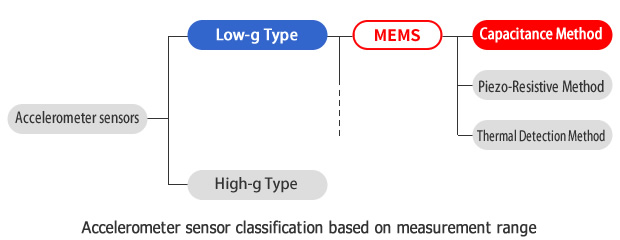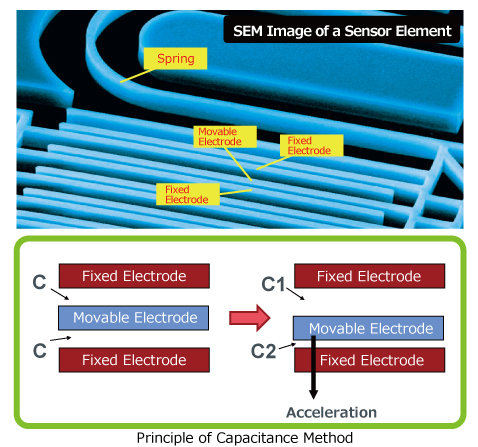# ＜Accelerometer sensors＞

## What is an accelerometer sensor?

Accelerometer sensors are ICs that measure acceleration, which is the change in speed (velocity) per unit time.
Measuring acceleration makes it possible to obtain information such as object inclination and vibration.
M/s2 is the international (SI*) unit for acceleration.
g is also used as a unit for acceleration, relative to standard gravity (1g = 9.80665m/s2).
Other units include Gal (CGS) used to measure seismic acceleration.

*SI: International System of Units (Système international d'unités)
An international unit expressed in units of length (m), mass (kg), and time (s) [MKS].

*Standard Gravity
Acceleration of an object caused by gravity. Represents the acceleration per unit time an object falls when dropped (9.80665m/s2).

※ Gal
CGS system (length cm, mass g, time s) for acceleration. This is defined as 1/100 of the SI system (1 Gal=0.01m/s2).
Accelerometer sensors are generally classified as low-g and high-g types as shown below.### Capacitance-type accelerometers

ROHM Group accelerometers are capacitive types developed using MEMS technology.
The sensor element consists of a fixed electrode comprised of Si, a working electrode, and spring. In a state with no acceleration, the distance between the fixed and movable electrode is the same. When acceleration is applied, the movable electrode is displaced. This causes a change in the positional relationship with the fixed electrode, changing the capacitance between the electrodes. The capacitance change is converted to voltage by an ASIC* and used to calculate acceleration.